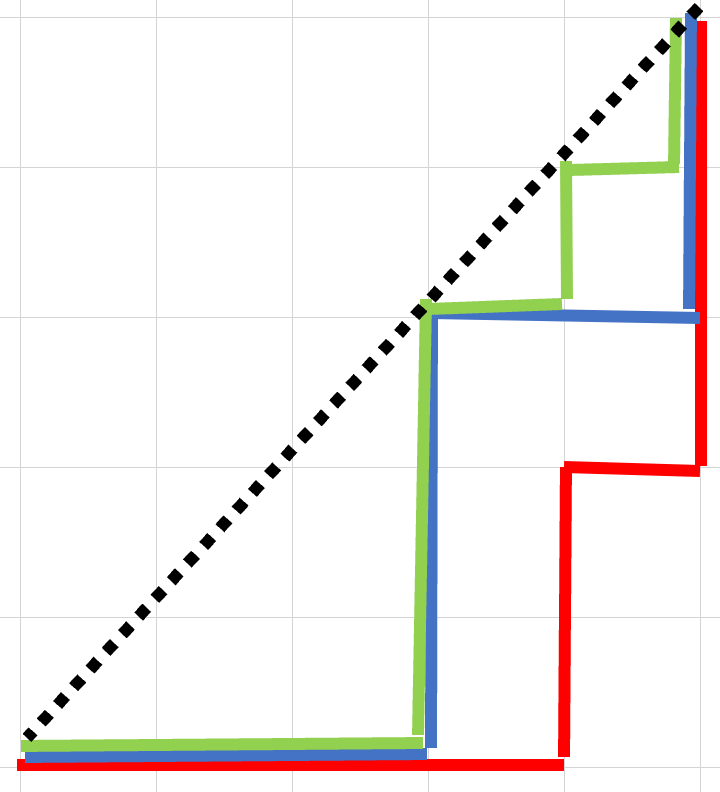……就算是单程票，也是可以的吧？

0%

# 引入

## 杨图，杨表，勾长公式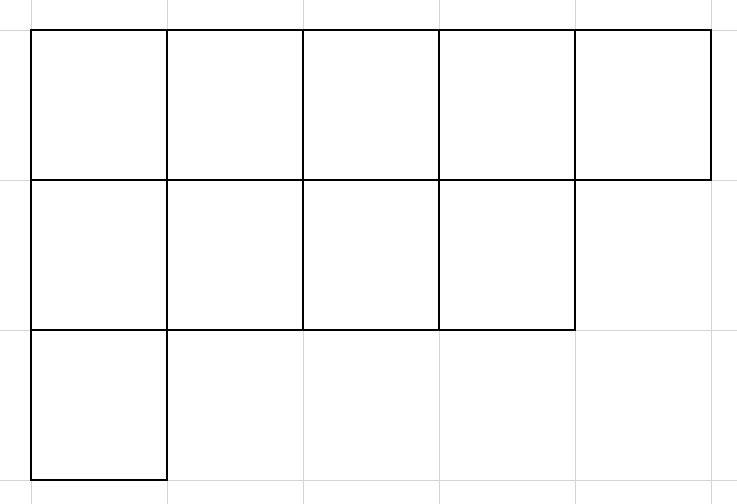## 斜杨图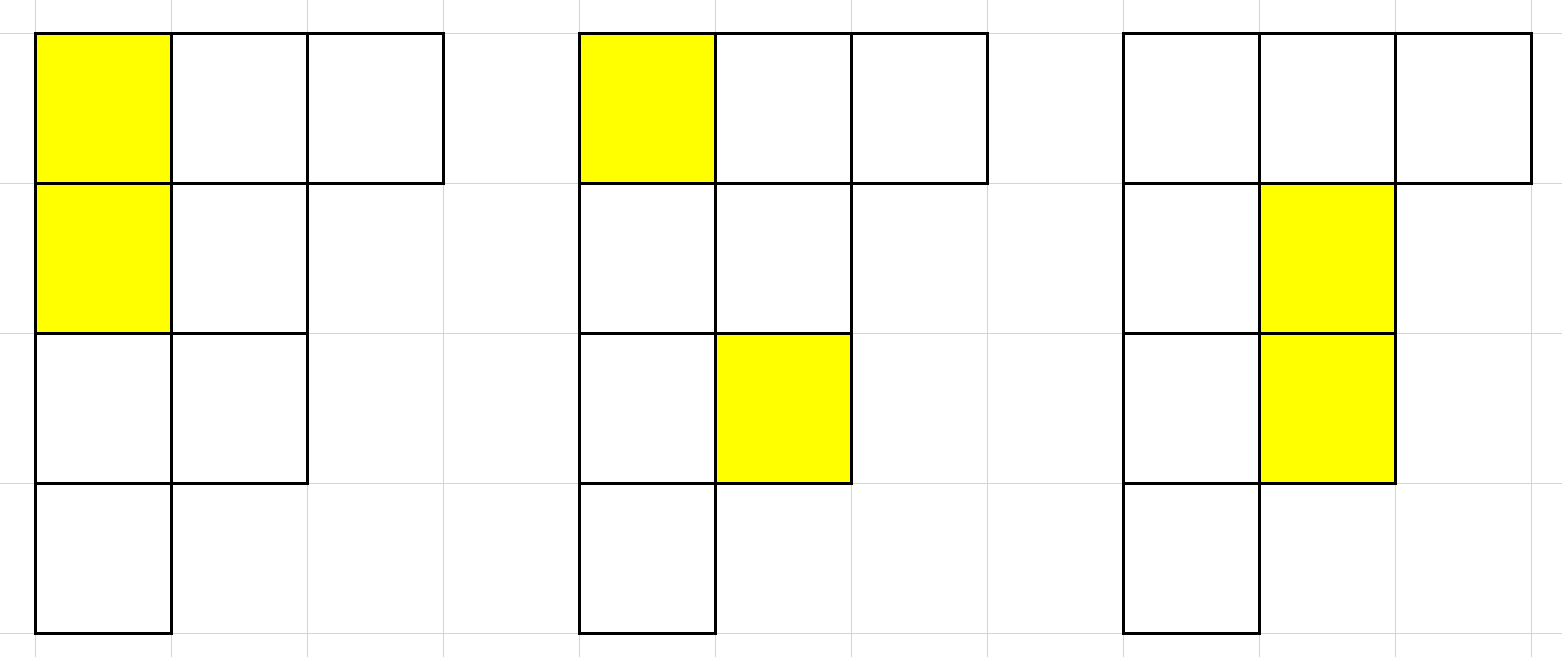# RSK 算法，杨表与 LIS 问题

## RSK 算法

### 行插入

• 找到本行最小的比 $x$ 大的数 $y$。如果找不到这样的 $y$，则把 $x$ 放在本行末尾并结束算法。
• 交换 $x,y$。将 $y$ 行插入下一行。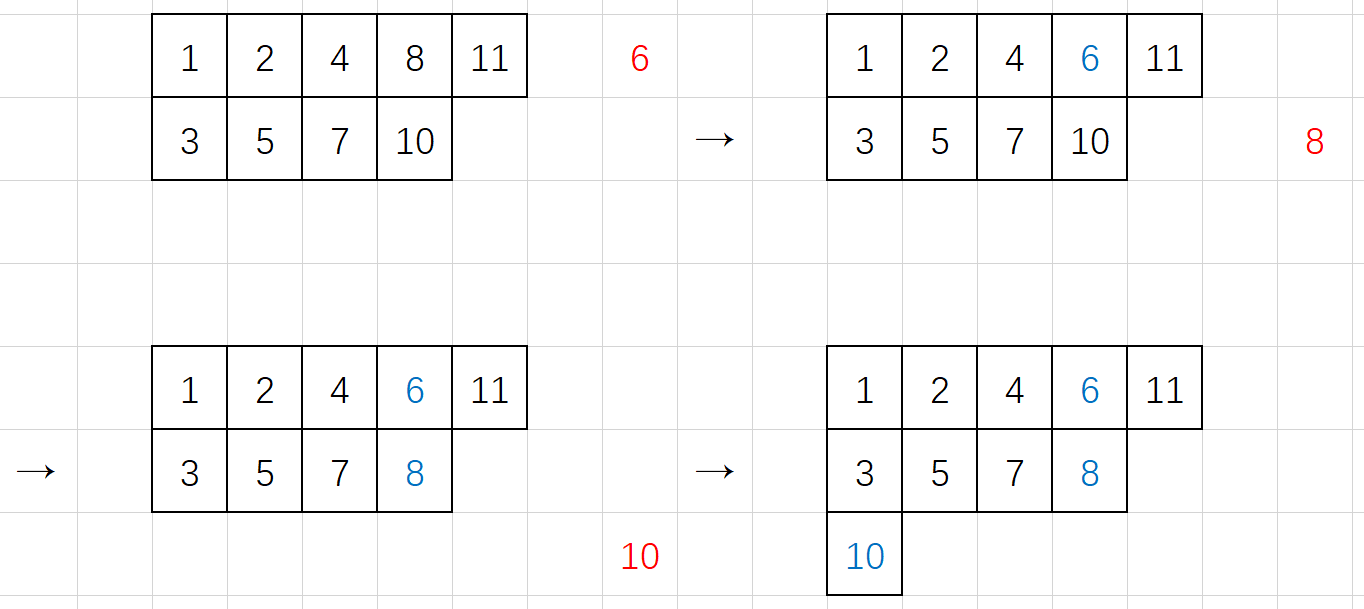### 删除

• 如果这是第一行，结束算法。

• 找到上一行最大的比 $x$ 小的数 $y$（显然一定存在）

• 交换 $x,y$，移到上一行继续算法。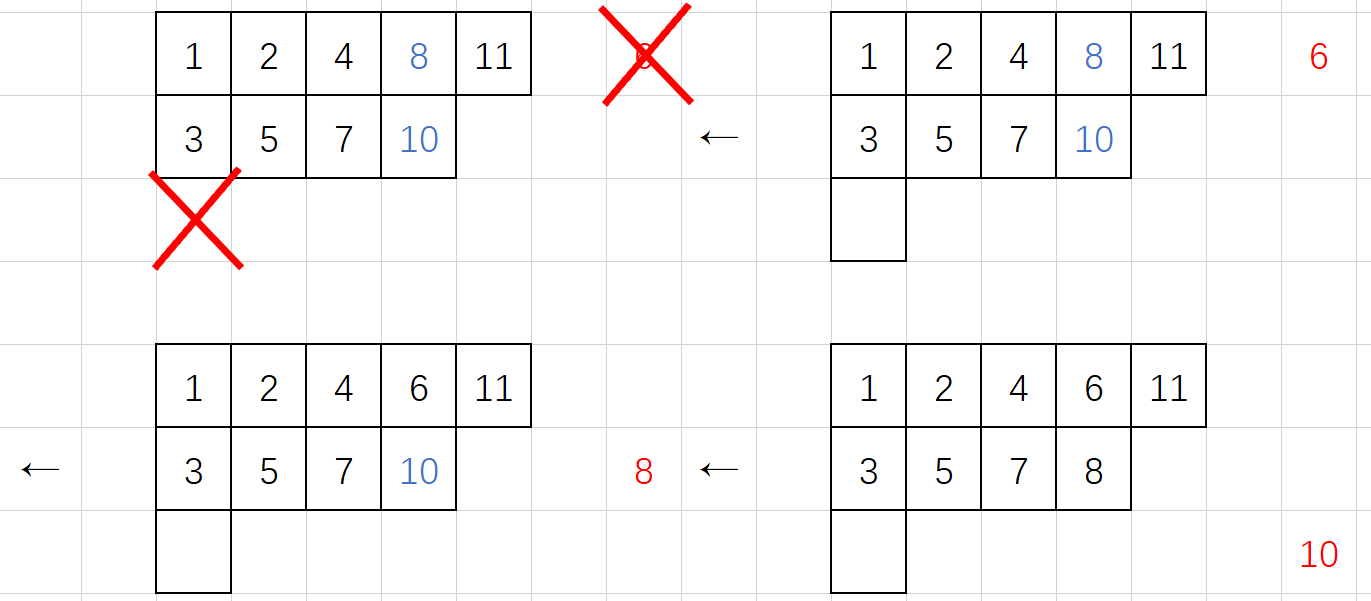## 杨表和 LIS 问题

LIS 即最长上升子序列，LDS 即最长下降子序列。

• 杨表第一行的长度即 $s$ 的 LIS 的长度。但是第一行并非 $s$ 的 LIS。

• 若有 $s\rightarrow(P,Q)$，则 $s$ 的翻转 $\text{rev}(s)\rightarrow(P^T,Q’)$。其中 $P^T$ 是 $P$ 的转置，$Q’$ 和 $Q$ 没什么关系。

• 于是我们得到杨表第一列的长度即 $s$ 的 LDS 的长度。

# 杨表和网格图路径数

## 卡塔兰数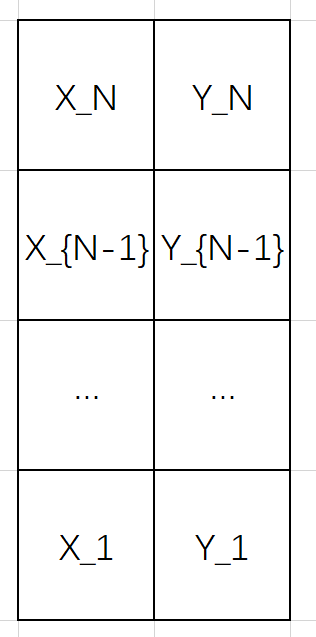## 「雅礼集训 2017 Day11」PATH

FFT 统计有多少对 $(i,j)$ 满足 $a_i-i-a_j+j=k$，然后就好了。

## LGV 引理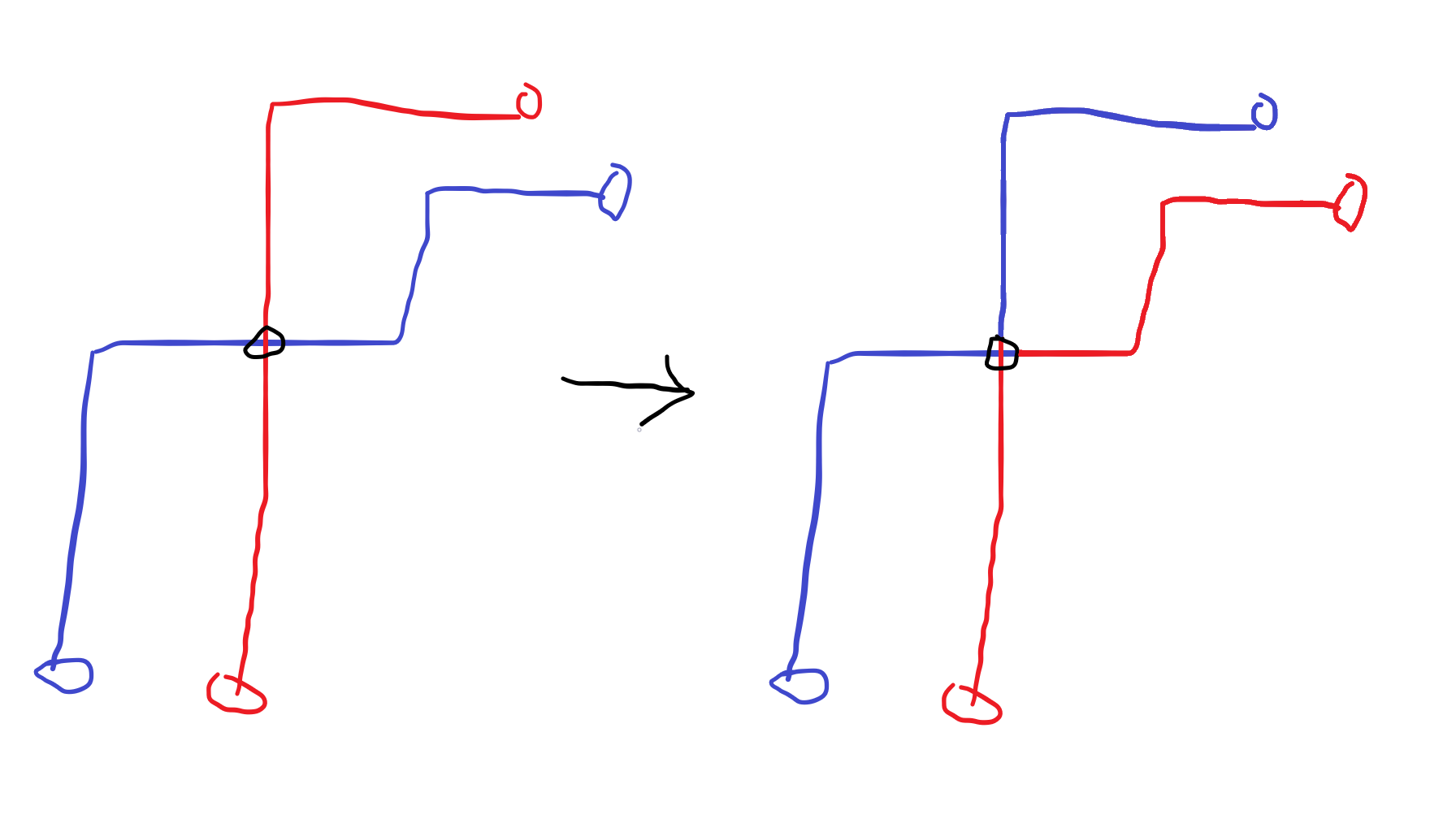## k-Dyck Path

1-Dyck Path 就是卡塔兰路径。k-Dyck Path 是 $k$ 条卡塔兰路径，但第二条必须完全在第一条“内部”，第三条必须完全在第二条内部……